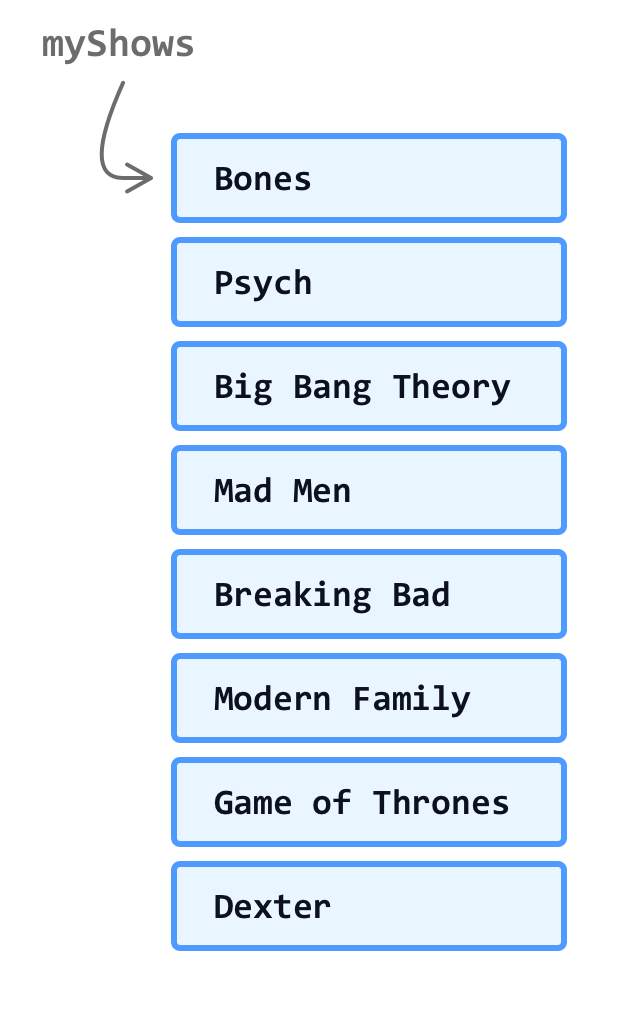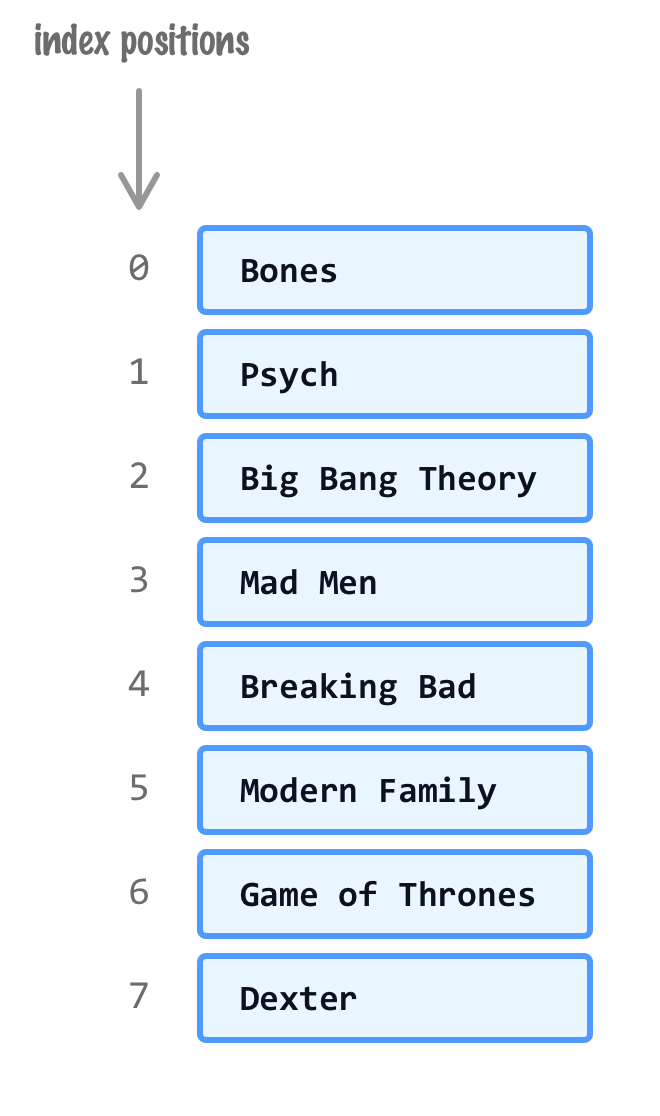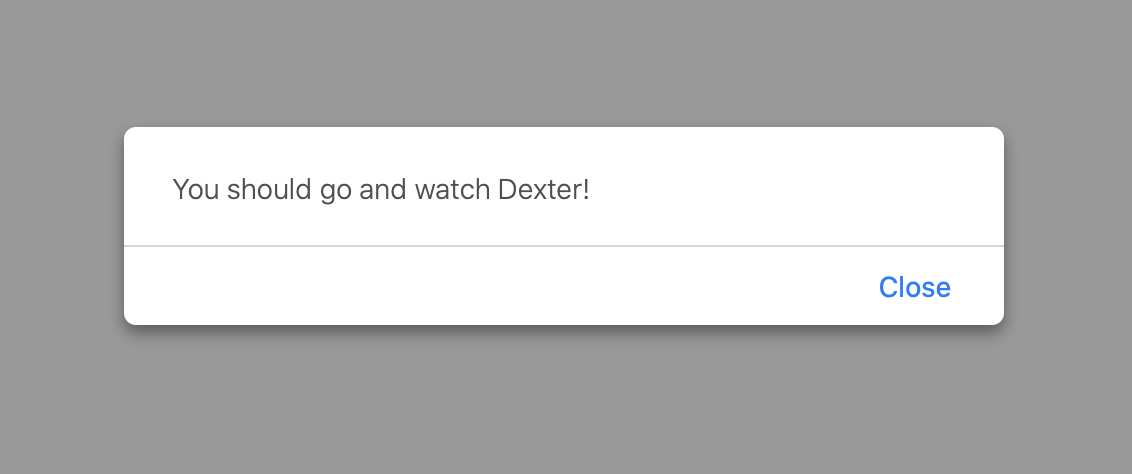# Picking a Random Item from an Array

by kirupa   |   filed under Arrays

Using just a single line of code, learn how to randomly pick an item from an array.

Let's say we have an array called myShows that contains a list of some popular TV shows that you may like to watch:Here is the problem. With so many great shows, how do you know what you want to watch at any given time? Unfortunately, you are unable to pick a show and stick with it beyond the first few seconds of the opening credits. Your friends all give different answers. Your parents haven't even heard of these shows, so they can't help you out much. Your psychiatrist stopped returning your calls. You are stuck.

Fortunately, whenever I find myself in a situation where I am all alone and having to make a difficult choice, I do what I do best. I put all of my choices into an array and write some JavaScript to randomly pick a choice - a choice that I unquestioningly follow. In this article, we will learn how to write this JavaScript ourselves. We will learn how to write some code to randomly pick an item from array filled with items!

Onwards!

#### OMG! An Arrays Book Written by Kirupa?!!

To kick your array skills into the stratosphere, everything you need to be an arrays expert is available in both paperback and digital editions.

## Code for Picking a Random Array Value

Let's jump right to it. The code for picking a random value from an array looks as follows:

``let randomValue = myArray[Math.floor(Math.random() * myArray.length)];``

Replace myArray with the name of the variable that actually stores your array. That's it. To see this as part of an example, we first need an array:

``````let myShows = ['Bones', 'Psych', 'Big Bang Theory', 'Mad Men',
'Breaking Bad', 'Modern Family', 'Game of Thrones', 'Dexter'];
``````

Our array is called myShows, so using the code we saw earlier earlier, the way we pick a random value from this array is by doing this:

``let show = myShows[Math.floor(Math.random() * myShows.length)];``

If you run this code, our show variable will store the name of a randomly picked show from your myShows array.

## How This All Works

The technique behind making our one line of code work requires only a slight understanding of arrays and how to work with random numbers. We will start with the random numbers angle first. The basic formula for picking a random number between a range of numbers is:

``Math.floor(Math.random() * (1 + High - Low)) + Low;``

All we need is a high number and a low number to define the range of numbers we want. This code will randomly (and fairly!) pick a random number between our range. The details of this are outlined in the Random Numbers tutorial, so check that out later if you want to go deeper into the world of random numbers.

What is the high number and the low number in our case? Since we are dealing with arrays, we know that the position of an item in an array is based on an index number. This index number starts at 0 and increments by one until we hit the last item in our array:The low number for us will be 0. This corresponds to the first item in our array. The formula right now will look as follows:

``Math.floor(Math.random() * (1 + High));``

The high number is the last item in our array. The index position of the last item in your array can be found by taking the length of our array and subtracting that value by 1:

``let lastItem = myArray.length - 1;``

If we substitute myArray.length - 1 into the high variable in our formula, here is what we would get:

``Math.floor(Math.random() * (1 + myArray.length - 1));``

The 1's will cancel out leaving our random index position to be:

``Math.floor(Math.random() * myArray.length);``

Getting the index position takes us most of the way there. We will use this index position to retrieve the value associated with it in our array:

``let value = myArray[Math.floor(Math.random() * myArray.length)];``

Replace myArray with myShows to get the exact syntax we saw a few hundred pixels earlier.

## Conclusion

So there you have it. By simply combining our knowledge of arrays and random numbers, we can create a single line of code that randomly picks a value from an array. In case you were wondering, here is what the script told me to watch:I guess that is what I am going to do for the next few hours. See you all later!

Got a question or just want to chat? Comment below or drop by our forums (they are actually the same thing!) where a bunch of the friendliest people you'll ever run into will be happy to help you out!